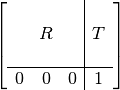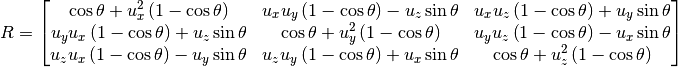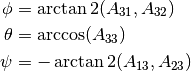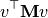# utils Package¶

## containers Module¶

class Queue[source]

Bases: object

FIFO

clear()[source]

Remove all elements of the queue

convert_to_list()[source]

Return the elements in an ordered list

count()[source]
is_empty()[source]
pull()[source]

Remove and return the first element of the queue

put(element)[source]

Appends the element as the last object of the queue

## generic Module¶

Generic utility functions to

• write variables and tuples to file
• write and read setting from a file
• modify and create tuples.
get_current_epoch_time()[source]

Return the current time in seconds since the Epoch.

Fractions of a second may be present if the OS provides them (UNIX-like do).

Returns: number of seconds since the Epoch float
insert_in_tuple(tuple_, index, item_)[source]
nested_iterable_to_tuple(iterable_)[source]
read_settings(filename, section)[source]

Read section from settings file at filename.

Parameters: filename (str) – settings file section (str) – settings section settings section dictionary dict

Example:

>>> read_settings('test.cfg', 'mySection')
{'a_key': 1.1111, 'another_key': False}

write_settings(filename, section, mapped_values)[source]

Write mapped_values at section in settings file at filename.

Parameters: filename (str) – settings file section (str) – settings section mapped_values (dict) – values to write

Example:

>>> write_settings('test.cfg', 'mySection', {'a_key': 1.1111, 'another_key': False})

write_tuple_to_file(text_file, tuple_)[source]
write_var_to_file(text_file, var)[source]

## geometry Module¶

class Transform(pos=None, rot=None)[source]

Bases: object

An homogeneous transform.

It is a composition of rotation and translation. Mathematically it can be expressed aswhere R is the 3x3 submatrix describing rotation and T is the 3x1 submatrix describing translation.

Constructor.

With empty arguments it’s just a 4x4 identity matrix.

Parameters: pos (tuple, numpy.ndarray or None) – a size 3 vector, or 3x1 or 1x3 matrix rot (tuple, numpy.ndarray or None) – 3x3 or 9x1 rotation matrix
get_long_tuple()[source]
get_rotation(as_numpy=False)[source]

Get the rotation component (matrix).

Parameters: as_numpy – whether to return a numpy object or a tuple 3x3 rotation matrix tuple of tuples or numpy.ndarray
get_translation(as_numpy=False)[source]

Get the translation component (vector).

Parameters: as_numpy – whether to return a numpy object or a tuple 3-sequence tuple or numpy.ndarray
matrix

Return matrix that contains the transform values.

Returns: 4x4 matrix numpy.ndarray
calc_compass_angle(rot)[source]

Return the angle around the vertical axis with respect to the X+ axis, i.e. the angular orientation inherent of a rotation matrix rot, constrained to the plane aligned with the horizon (XZ, since the vertical axis is Y).

calc_inclination(rot)[source]

Return the inclination (as pitch and roll) inherent of rotation matrix rot, with respect to the plane (XZ, since the vertical axis is Y). pitch is the rotation around Z and roll around Y.

Examples:

>>> rot = calc_rotation_matrix((1.0, 0.0, 0.0), pi/6)
>>> pitch, roll = gemut.calc_inclination(rot)
0.0, pi/6

>>> rot = calc_rotation_matrix((0.0, 1.0, 0.0), whatever)
>>> pitch, roll = gemut.calc_inclination(rot)
0.0, 0.0

>>> rot = calc_rotation_matrix((0.0, 0.0, 1.0), pi/6)
>>> pitch, roll = gemut.calc_inclination(rot)
pi/6, 0.0

calc_rotation_matrix(axis, angle)[source]

Return the row-major 3x3 rotation matrix defining a rotation of magnitude angle around axis.

Formula is the same as the one presented here (as of 2011.12.01): http://goo.gl/RkW80The returned matrix format is length-9 tuple.

get_body_relative_vector(body, vector)[source]

Return the 3-vector vector transformed into the local coordinate system of ODE body ‘body’

make_OpenGL_matrix(rot, pos)[source]

Return an OpenGL compatible (column-major, 4x4 homogeneous) transformation matrix from ODE compatible (row-major, 3x3) rotation matrix rotation and position vector position.

The returned matrix format is length-9 tuple.

rot_matrix_to_euler_angles(rot)[source]

Return the 3-1-3 Euler angles phi, theta and psi (using the x-convention) corresponding to the rotation matrix rot, which is a tuple of three 3-element tuples, where each one is a row (what is called row-major order).

Using the x-convention, the 3-1-3 Euler angles phi, theta and psi (around the Z, X and again the Z-axis) can be obtained as follows:http://en.wikipedia.org/wiki/Rotation_representation_(mathematics)%23Rotation_matrix_.E2.86.94_Euler_angles

rot_matrix_to_hom_transform(rot)[source]

Convert a rotation matrix to a homogeneous transform.

source: transform.r2t in Corke’s Robotic Toolbox (python)

Parameters: rot (a tuple, a tuple of tuples or numpy.ndarray) – 3x3 rotation matrix

## mathematical Module¶

Functions to perform operations over vectors and matrices; deal with homogeneous transforms; convert angles and other structures.

acos_dot3(vector1, vector2)[source]

Return the angle between unit 3-dimension vector1 and vector2.

add3(vector1, vector2)[source]

Return the sum of 3-dimension vector1 and vector2.

calc_acceleration(time_step, vel0, vel1)[source]

Calculate the vectorial substraction vel1 - vel0 divided by time step. If any of the vectors is None, then None is returned.

vel1 is the velocity measured time_step seconds after vel0.

cross_product(vector1, vector2)[source]

Return the cross product of 3-dimension vector1 and vector2.

degrees_to_radians(degrees_)[source]
dist3(vector1, vector2)[source]

Return the distance between point 3-dimension vector1 and vector2.

div_by_scalar3(vector, scalar)[source]

Return 3-dimension vector divided by scalar.

dot_product(vec1, vec2)[source]

Efficient dot-product operation between two vectors of the same size. source: http://docs.python.org/library/itertools.html

dot_product3(vector1, vector2)[source]

Return the dot product of 3-dimension vector1 and vector2.

length2(vector)[source]

Return the length of a 2-dimension vector.

length3(vector)[source]

Return the length of a 3-dimension vector.

matrix_as_3x3_tuples(tuple_9)[source]

Return tuple_9 as a 3-tuple of 3-tuples.

Parameters: tuple_9 – tuple of 9 elements tuple_9 formatted as tuple of tuples
matrix_as_tuple(matrix_)[source]

Convert matrix_ to a tuple.

Example:

>>> matrix_as_tuple(((1, 2), (3, 4)))
(1, 2, 3, 4)

Parameters: matrix (tuple) – nested tuples matrix_ flattened as a tuple tuple
matrix_multiply(matrix1, matrix2)[source]

Return the matrix multiplication of matrix1 and matrix2.

Parameters: matrix1 – LxM matrix matrix2 – MxN matrix LxN matrix, product of matrix1 and matrix2 tuple of tuples
mult_by_scalar3(vector, scalar)[source]

Return 3-dimension vector multiplied by scalar.

neg3(vector)[source]

Return the negation of 3-dimension vector.

norm3(vector)[source]

Return the unit length vector parallel to 3-dimension vector.

np_matrix_to_tuple(array_)[source]

Convert Numpy 2D array (i.e. matrix) to a tuple of tuples.

Example:

>>> arr = numpy.array(((2, 2), (2, -2)))
>>> np_matrix_to_tuple(arr)
((2, 2), (2, -2))

Parameters: array (numpy.ndarray) – 2D array (i.e. matrix) matrix as tuple of tuples
project3(vector, unit_vector)[source]

Return projection of 3-dimension vector onto unit 3-dimension unit_vector.

radians_to_degrees(radians_)[source]
rotate3(rot, vector)[source]

Return the rotation of 3-dimension vector by 3x3 (row major) matrix rot.

sign(x)[source]

Return 1.0 if x is positive, -1.0 otherwise.

sub3(vector1, vector2)[source]

Return the difference between 3-dimension vector1 and vector2.

transpose3(matrix)[source]

Return the inversion (transpose) of 3x3 rotation matrix matrix.

unitize(vector_)[source]

Unitize a vector, i.e. return a unit-length vector parallel to vector.

vec3_degrees_to_radians(vector_)[source]
vec3_radians_to_degrees(vector_)[source]
vector_matrix_vector(vector_, matrix_)[source]

Return the product of vector_ transposed, matrix_ and vector again, which is a scalar value.z_axis(rot)[source]

Return the z-axis vector from 3x3 (row major) rotation matrix rot.

## version Module¶

get_hg_changeset()[source]

Return the global revision id that identifies the working copy.

To obtain the value it runs the command hg identify --id, whose short form is hg id -i.

>>> get_hg_changeset()
1a4b04cf687a
>>> get_hg_changeset()
1a4b04cf687a+


Note

When there are outstanding (i.e. uncommitted) changes in the working copy, a + character will be appended to the current revision id.

get_hg_tip_timestamp()[source]

Return a numeric identifier of the latest changeset of the current repository based on its timestamp.

To obtain the value it runs the command hg tip --template '{date}'

>> get_hg_tip_timestamp() ‘20130328021918’

Based on: django.utils.get_git_changeset @ commit 9098504, and http://hgbook.red-bean.com/read/customizing-the-output-of-mercurial.html

get_version(version=None, length=u'full')[source]

Return a PEP 386-compliant version number from version.

Parameters: version (tuple of strings) – the value to format, expressed as a tuple of strings, of length 5, with the element before last (i.e. version) equal to any of the following: ('alpha', 'beta', 'rc', 'final') length (basestring) – the format of the returned value, equal to any of the following: ('short', 'medium', 'full') version as a string str
>>> get_version(version=(0, 4, 0, 'alpha', 0))
0.4.dev20130401011455
>>> get_version(version=(0, 4, 0, 'alpha', 1))
0.4a1
>>> get_version(version=(0, 4, 1, 'alpha', 0))
0.4.1.dev20130401011455
>>> get_version(version=(0, 4, 1, 'alpha', 1))
0.4.1a1
>>> get_version(version=(0, 4, 0, 'beta', 0))
0.4b0
>>> get_version(version=(0, 4, 0, 'rc', 0))
0.4c0
>>> get_version(version=(0, 4, 0, 'final', 0))
0.4
>>> get_version(version=(0, 4, 0, 'final', 1))
0.4
>>> get_version(version=(0, 4, 1, 'final', 0))
0.4.1

>>> get_version(version=(0, 4, 0, 'alpha', 0), length='medium')
0.4.dev
>>> get_version(version=(0, 4, 0, 'alpha', 0), length='short')
0.4


Based on: django.utils.version @ commit 9098504. Django’s license is included at docs/Django BSD-LICENSE.txt